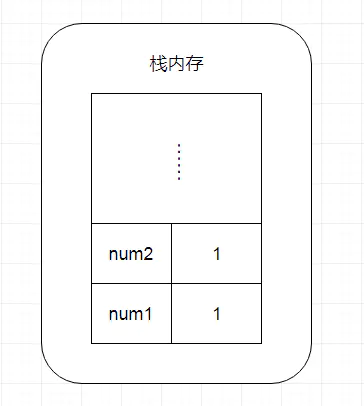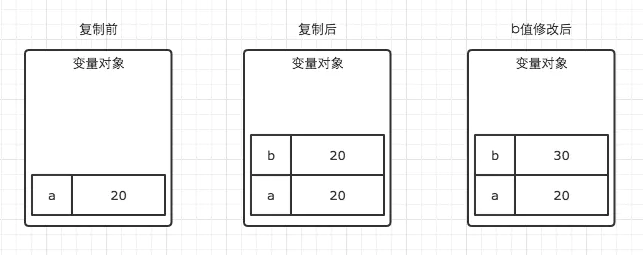# # JS中的栈内存堆内存

## # 引言

JS的内存空间分为栈(stack)、堆(heap)、池(一般也会归类为栈中)。

## # 栈数据结构## # 变量类型与内存的关系

### # 基本数据类型

1. Sting
2. Number
3. Boolean
4. null
5. undefined
6. Symbol

``````let num1 = 1;
let num2 = 1;
``````
1
2PS: 需要注意的是闭包中的基本数据类型变量不保存在栈内存中，而是保存在堆内存中。这个问题，我们后文再说。

### # 引用数据类型

Array,Function,Object...可以认为除了上文提到的基本数据类型以外，所有类型都是引用数据类型。

``````// 基本数据类型-栈内存
let a1 = 0;
// 基本数据类型-栈内存
let a2 = 'this is string';
// 基本数据类型-栈内存
let a3 = null;

// 对象的指针存放在栈内存中，指针指向的对象存放在堆内存中
let b = { m: 20 };
// 数组的指针存放在栈内存中，指针指向的数组存放在堆内存中
let c = [1, 2, 3];
``````
1
2
3
4
5
6
7
8
9
10
11## # 从内存角度来看变量复制

### # 基本数据类型的复制

``````let a = 20;
let b = a;
b = 30;
console.log(a); // 此时a的值是多少，是30？还是20？
``````
1
2
3
4### # 引用数据类型的复制

``````let m = { a: 10, b: 20 };
let n = m;
n.a = 15;
console.log(m.a) //此时m.a的值是多少，是10？还是15？
``````
1
2
3
4